# Python编程入门：基础语法详解

Python是一种计算机程序设计语言。是一种动态的、面向对象的脚本语言。今天给大家分享Python编程入门教程之基础语法详解，接下来一起来看吧！1.内置的变量类型：

Python是有变量类型的，而且会强制检查变量类型。内置的变量类型有如下几种：

#浮点

float_number = 2.3

#复数

complex_number = 1 + 5j

#整型

integer_number = 1

#list 序列

sample_list = [2,52,36,'abc']

#嵌套

sample_nest = [(2,4,6),{5:7,9:11,'key':[2,5]},6]

#dictionary 字典

sample_dic = {"key":value, 5:10}

#tuple 只读的序列

sample_tuple = (3,9,"ab")

2.字符串

python中声明一个字符串通常有三种方法，''、" "和''' '''，这三种方法在声明普通字符串时的效果是完全一样的，区别在于字符串本身中存在引号的情况，举例如下：

word = 'good'

sentence = "hello world"

paragraph = '''good noon:"nice to meet you."'''

python中单行注释采用#开头。

#!/usr/bin/python

# First comment

print "Hello, world!"; # second comment

'''This is a comment.

This is a comment, too.

This is a comment, too.

python允许处理unicode字符串，加前缀u或U， 如 u"this is an unicode string"。

3.Python标识符

python中的标识符是区分大小写的。

4.Python保留字符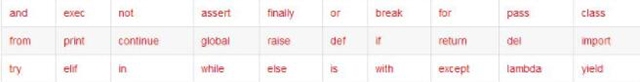5.行和缩进

if True:

print "True"

else:

print "False"

if True:

print "True"

else:

print "False"

python中分号;标识一个逻辑行的结束，但是实际中一般每个物理行只写一个逻辑行，可以避免使用分号。这样书写便于阅读。注意：不要混合使用空格和制表符来缩进，因为在跨越不同的平台时无法正常工作。

s = "peter is

1. 运算符与其用法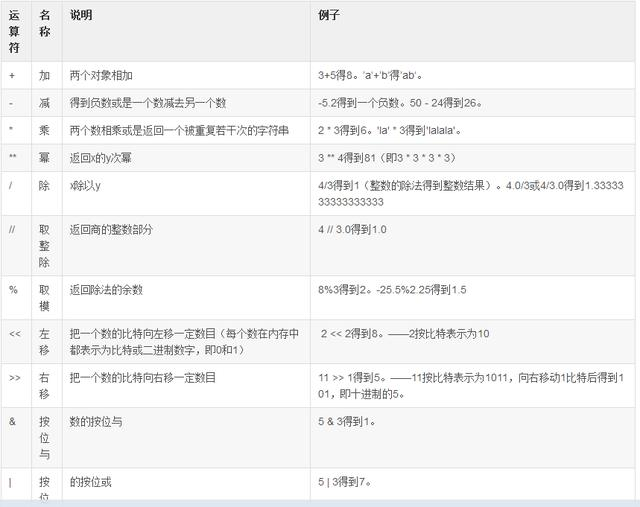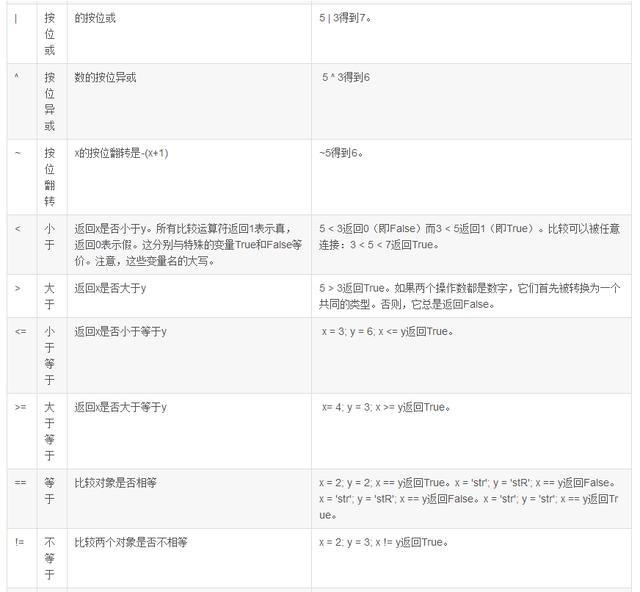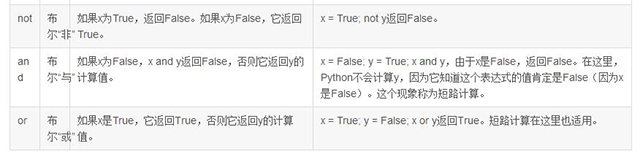2. 运算符优先级（从低到高）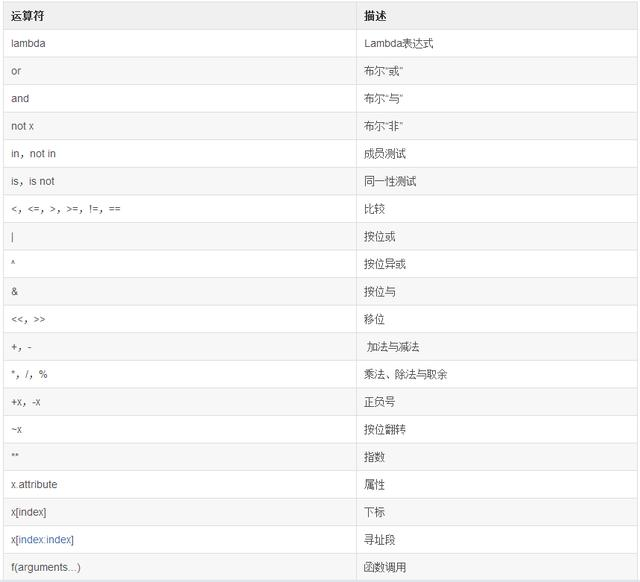1. 条件语句

1.1 if语句

a = input("a:")

b = input("b:")

if(a > b):

print a, " > ", b

if else语句：

a = input("a:")

b = input("b:")

if(a > b):

print a, " > ", b

else:

print a, " < ", b

1.2 if…elif…else语句

score = raw_input("score:")

score=int(score)

if(score >= 90) and (score <= 100):

print "A"

elif(score >= 80) and (score < 90):

print "B"

elif(score >= 60) and (score < 80):

print "C"

else:

print "D"

raw_input() 读取输入值。

1.3 if语句的嵌套

x = -1

y = 99

if(x >= 0):

if(x > 0): #嵌套的if语句

y = 1

else:

y = 0

else:

y = -1

print "y =", y

1.4 实现switch语句的功能

python中没有switch特殊字，Python可以通过字典实现switch语句的功能。

from __future__ import division

x = 1

y = 2

operator = "/"

result = {

"+" : x + y,

"-" : x - y,

"*" : x * y,

"/" : x / y

}

print result.get(operator)

a) 创建一个switch类，该类继承自Python的祖先类object。调用构造函数init( )初始化需要匹配的字符串，并需要定义两个成员变量value和fall。Value用于存放需要匹配的字符串，fall用于记录是否匹配成功，初始值为false，标识匹配不成功。如果匹配成功，程序往后执行。

b) 定义一个match( )方法，该方法用于用于匹配case子句。这里需要考虑三种情况：首先是匹配成功的情况，其次是匹配失败的默认case子句，最后是case子句中没有使用break中断的情况。

c) 重写__iter__( )方法，定义该方法后才能使switch类用于循环语句中。__iter__( )调用match( )方法进行匹配。通过yield保留字，使函数可以在循环中迭代。此外，调用StopIteration异常中断循环。

d) 编写调用代码，在for…in…循环中使用switch类。

#!/usr/bin/python

# -*- coding: UTF-8 -*-

class switch(object):

def __init__(self, value): # 初始化需要匹配的值value

self.value = value

self.fall = False # 如果匹配到的case语句中没有break，则fall为true。

def __iter__(self):

yield self.match # 调用match方法 返回一个生成器

raise StopIteration # StopIteration 异常来判断for循环是否结束

def match(self, *args): # 模拟case子句的方法

if self.fall or not args: # 如果fall为true，则继续执行下面的case子句

# 或case子句没有匹配项，则流转到默认分支。

return True

elif self.value in args: # 匹配成功

self.fall = True

return True

else: # 匹配失败

return False

operator = "+"

x = 1

y = 2

for case in switch(operator): # switch只能用于for in循环中

if case('+'):

print x + y

break

if case('-'):

print x - y

break

if case('*'):

print x * y

break

if case('/'):

print x / y

break

if case(): # 默认分支

print ""

2.while...语句

while True:

pass

else:

pass

#else语句可选，当while为False时，else语句被执行。 pass是空语句。

3.for 循环

for i in range(0, 5):

print i

else:

pass

# 打印0到4

def sumOf(a, b):

return a + b

4.1 局部变量

def func():

global x

print "x is ", x

x = 1

x = 3

func()

print x

3

1

4.2 默认参数

def say(msg, times = 1):

print msg * times

say("peter")

say("peter", 3)

4.3 关键参数

def func(a, b=2, c=3):

print "a is %s, b is %s, c is %s" % (a, b, c)

func(1) #输出a is 1, b is 2, c is 3

func(1, 5) #输出a is 1, b is 5, c is 3

func(1, c = 10) #输出a is 1, b is 2, c is 10

func(c = 20, a = 30) #输出a is 30, b is 2, c is 20

4.4 return语句

return语句用来从一个函数返回，即跳出函数。可从函数返回一个值。